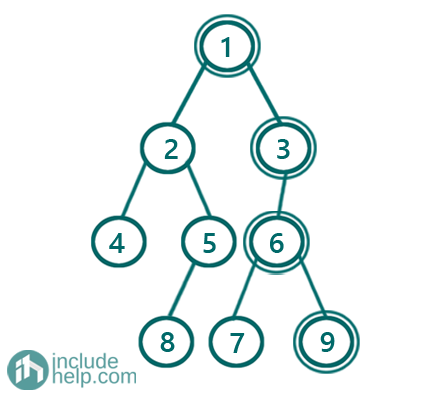# Sum of nodes on the longest path from the root to the leaf node

In this article, we are going to see how to find the sum of the longest path in root to leaf path?
Submitted by Radib Kar, on August 28, 2020

In this article, we are going to see how to find the sum of the longest path from the root to leaf path? If there is more than one longest path then pick up the one which has the greatest sum.

In the below example,We have the following paths which all are the longest ones.

[1-> 2-> 5-> 8] //sum=16
[1-> 3-> 6-> 7] //sum=17
[1-> 3-> 6-> 9] //sum=19

So, the output will be 19 (sum of the marked path)

Solution:

Here, we use recursion to solve the problem. Here, we track both path length and sum. Below is the algorithm. To track both we keep them as pair <path length, sum>

1. Base case:
1. if root is NULL then return <0,0>, path length 0, sum = 0
2. if root is leaf then return <1,root value>, path length 1, sum =root->value
2. Find recursively the <path length, sum> pair from both the subtrees. Say l is the result for the left subtree, r is the result for the right subtree.
```If path length from left subtree> path length from right subtree
Choose the left subtree path and
return <length of the path from left subtree+1, sum from left subtree + root value>
Else if path length from left subtree < path length from right subtree
Choose the right subtree path and
return <length of the path from right subtree+1, sum from right subtree + root value>
Else if both subtree path length is the same Then,
Pick one which has maximum sum and
return <subtree path length +1, max sum from subtrees +root value>
```

C++ Implementation:

```#include <bits/stdc++.h>
using namespace std;

// tree node is defined
class TreeNode {
public:
int val;
TreeNode* left;
TreeNode* right;
TreeNode(int data)
{
val = data;
left = NULL;
right = NULL;
}
};

//recursion which returns longest root path sum
//pair structure: <path length, sum of the path>
pair<int, int> sumOfLongRootToLeafPathRecur(TreeNode* root)
{
//base case
//1. if root is NULL then return <0,0>, path length 0, sum 0
if (!root)
return make_pair(0, 0);
//2. if root is leaf then return <1,root value>,
//path length 1, sum root->value
if (!root->left && !root->right)
return make_pair(1, root->val);

//for left subtree
pair<int, int> l = sumOfLongRootToLeafPathRecur(root->left);
//for right subtree
pair<int, int> r = sumOfLongRootToLeafPathRecur(root->right);

//if length of longest path in the
//left subtree > length of longest path in the right subtree
if (l.first > r.first) {
//return <length of path from left subtree+1, sum from left subtree + root val>
return make_pair(l.first + 1, l.second + root->val);
}
//if length of longest path in the
//left subtree < length of longest path in the right subtree
else if (l.first < r.first) {
//return <length of path from right subtree+1, sum from right subtree + root val>
return make_pair(r.first + 1, r.second + root->val);
}
else { //if both path length from subtrees are same pick max sum path from sum trees
if (l.second > r.second)
return make_pair(l.first + 1, l.second + root->val);
else
return make_pair(r.first + 1, r.second + root->val);
}
}

//function to return sum of longest path
int sumOfLongRootToLeafPath(TreeNode* root)
{

return sumOfLongRootToLeafPathRecur(root).second;
}

int main()
{
//building the tree like the example
TreeNode* root = new TreeNode(1);

root->left = new TreeNode(2);
root->right = new TreeNode(3);
root->left->left = new TreeNode(4);
root->left->right = new TreeNode(5);
root->right->left = new TreeNode(6);
root->left->right->left = new TreeNode(8);
root->right->left->left = new TreeNode(7);
root->right->left->right = new TreeNode(9);

cout << "Sum of the nodes in the longest root to leaf path: " << sumOfLongRootToLeafPath(root) << endl;

return 0;
}
```

Output:

```Sum of the nodes in the longest root to leaf path: 19
```

Languages: » C » C++ » C++ STL » Java » Data Structure » C#.Net » Android » Kotlin » SQL
Web Technologies: » PHP » Python » JavaScript » CSS » Ajax » Node.js » Web programming/HTML
Solved programs: » C » C++ » DS » Java » C#
Aptitude que. & ans.: » C » C++ » Java » DBMS
Interview que. & ans.: » C » Embedded C » Java » SEO » HR
CS Subjects: » CS Basics » O.S. » Networks » DBMS » Embedded Systems » Cloud Computing
» Machine learning » CS Organizations » Linux » DOS
More: » Articles » Puzzles » News/Updates